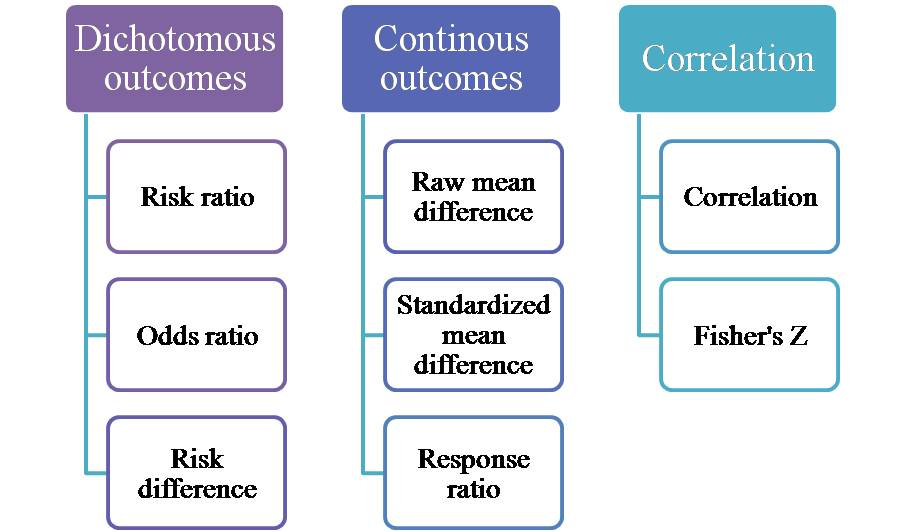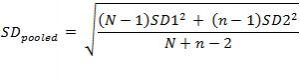# Computing meta analysis with different effect size with CMA

By Yashika Kapoor,Priya Chetty & Abhinash on October 31, 2017

The discussion surrounding meta-analysis has effect size at the prime spot. It is the magnitude or size of an effect and usually refers to the treatment effect in a comprehensive meta-analysis (CMA). For any given study the effect size cannot be constrained to a single index or measure. The article will introduce the concept of different effect size measures.  It will help to understand and choose appropriate effect size measures for analysing data. This is important because different studies generate different datasets of variable nature. They involve events or non-events or mean and standard deviation or other, where the measure of effect size also changes. To ensure selection of appropriate effect size:

1. Effect sizes from different studies should measure the same phenomenon.
2. The estimates should not require re-analyzing the raw data.
3. It should have sound technical characteristics.
4. The reported effect size should be significantly interpretable.

The figure below shows the different effect size measures computable in CMA, as determined by the different types of outcomes.Figure 1: Different types of effect size indices depending on the type of outcome

## Different outcomes of different effect size

Different study designs yield different outcomes, whether primary or secondary. As a result, while conducting a meta-analysis, it is essential to select the appropriate effect size measure. This will help the fulfilment of research goals and objectives. The different types of outcomes constitute continuous (mean and standard deviation), binary data, and correlations. The figure below shows the decision flow which could assist them in selecting the appropriate effect size for the study.

## The effect size for continuous outcomes

The CMA software allows for to calculation of the difference in means (Raw mean difference, unstandardized), the standard difference in means, Standard paired difference and Hedge’s g (Standardized mean differences), for continuous outcomes. If all the studies report results or outcomes over the same scale of measurement then the difference in means can be directly calculated. Whereas, if the studies report results or outcomes over different instruments, then the raw difference can be used.

In such scenarios, resort to the measures of standardized mean difference (SMD). The standardized measures include dividing the mean difference in each study by the respective standard deviation. This creates a standard index used to compare the outcomes across different studies. The important point of consideration arises with respect to the standard deviation while comparing the results from different study designs having different study groups.

### Standard deviation

In CMA specific formats demand standard deviation difference, while a common standard deviation of difference is required by others. The difference between the standard deviation of pre-post scores, within a single group is the standard deviation difference. Whereas the pooled standard deviation of the treatment and control groups (independent or unmatched) is a common standard deviation and is calculated using.The formula for standard deviation pooled for calculating effect size for comparison groups

Where;

• N = sample size of the first group,
• n = sample size of the second group.

The matched groups and one group (pre-post) designs also utilize standard deviation differences. Standard deviation difference calculation uses the following formula:The formula for standard deviation pooled for calculating effect size of single or matched groups

Note: The ‘log proportional change in the means of treatment and control group’ constitutes response ratio (Lajeunesse 2011). CMA does not offer the calculation of response ratio effect sizes. It is used when the outcome is measured on a physical scale such as length, weight, which it is unlikely to be zero. Response ratios are useful when the outcome has been measured on a ratio scale. These are not useful when the studies use non-natural units of scale, such as test scores because such scales do not have a natural zero point.

## The effect size for dichotomous outcome

When dealing with dichotomous outcomes, such as the number of events, ‘Risk ratio’, ‘Odds ratio’ or ‘Risk difference’ can be selected. CMA also allows to calculation of varied statistical versions of these classical effect measures, as shown in the figure below. In simple terms, risk ratio is the ratio of two risks and odds ratio is the ratio of two odds. The different measures of these ratios allow the drawing of statistical inferences of higher suitability or relevance. The figure below shows different measures.Figure 3: Variation of classical odds ratio, risk ratio and risk difference

## Effect size risk ratios

The odds ratio and risk ratio refer to a single strat or group and give information about the odds and risk of an event respectively (Byers et al 2014). However, a study design might consist of subgroups, such that the observations have been divided into two or four-fold tables, such as MH odds ratio for circumcised men having high ALEX scores and normal men having low ALEX scores (Morris & Waskett 2012).

In such a scenario, Mantel-Haenszel (MH) odds or risk ratio is found to be relevant. The MH ratio allows for the calculation of pooled odds or risk ratios across the strata of fourfold tables (Tripepi et al. 2010). Peto odds ratio allows the pooling of odds ratio from four-fold tables. It has a reputation for dealing with rare events (Bradburn et al. 2007).

Figure 3 above shows the logarithmic of classical and varied odds or risk ratios. The natural logs of ratios are better approximated by a normal distribution. The difference in the log parameters defines the ratios, on log scales. This aids in maintaining symmetry in the analysis (Balakrishnan 2014).

Risk difference (RD) computed using the raw data refers to the difference between the two risks. The computation of the difference using the raw units makes it an absolute measure and sensitive to baseline risks. Hence, RD is the preferred measure to report the clinical outcome of treatment. Also, the combination of RD from different strata gives MH RD (Klingenberg 2013).

## The effect size for correlation

The correlation variable can serve as an effect size measure for studies having one group only. Moreover, CMA offers another effect size measure, Fisher’s Z. Variance does not influence Fisher’s Z, like correlation.

The present article helps in selecting appropriate effect size measures in future meta-analyses. The next article will introduce manual data entry methods, through the means of the case study.

#### References

• Balakrishnan, N., 2014. Methods and applications of statistics in clinical trials, volume 1: concepts, principles, trials, and designs, John Wiley & Sons.
• Bradburn, M.J. et al., 2007. Much ado about nothing: a comparison of the performance of meta-analytical methods with rare events. Statistics in medicine, 26(1), pp.53–77.
• Byers, A.L. et al., 2014. Chronicity of posttraumatic stress disorder and risk of disability in older persons. JAMA psychiatry, 71(5), pp.540–546.
• Klingenberg, B., 2013. A new and improved confidence interval for the Mantel-Haenszel risk difference. Statistics in medicine, 33(17), pp.2968–2983.
• Lajeunesse, M.J., 2011. On the meta-analysis of response ratios for studies with correlated and multi-group designs. Ecology, 92(1), pp.2049–2055.
• Morris, B.J. & Waskett, J.H., 2012. A critique of Bollinger and Van Howe’s Alexithymia and Circumcision Trauma. A preliminary investigation. International Journal of Men’s Health, 11(2), pp.177–184.
• Tripepi, G. et al., 2010. Stratification for confounding – Part 1: The Mantel-Haenszel formula. Nephron Clinical Practice, 116(4), pp.317–321.